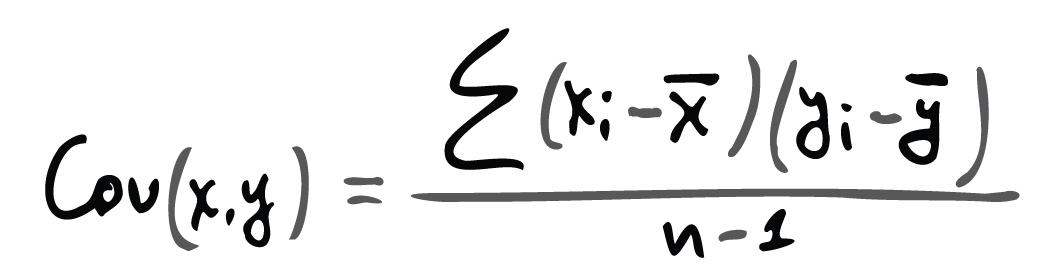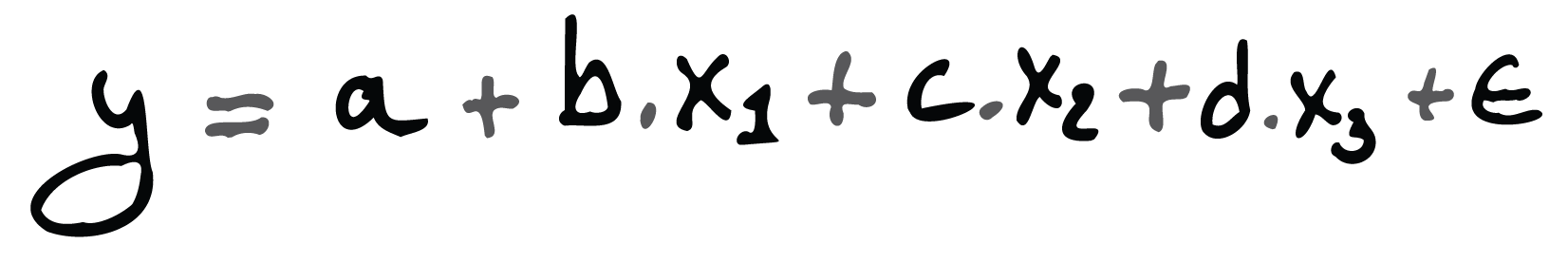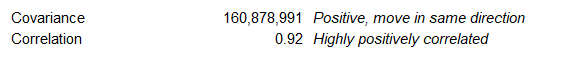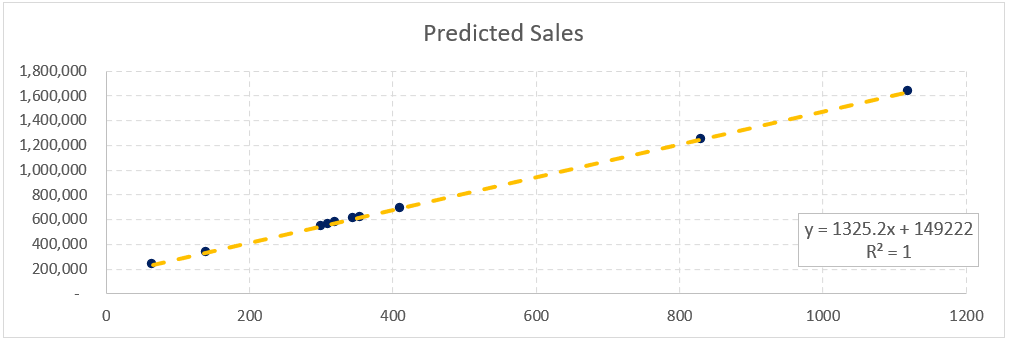## Regression Analysis in Financial Modeling

Regression Analysis represents a set of statistical methods and techniques, which we use to evaluate the relationship between variables. These are one dependent variable (our target) and one or more independent variables (predictors).

We have three primary variants of regression – simple linear, multiple linear, and non-linear. However, most of the time, we use linear regression models. Non-linear models are helpful when working with more complex data, where variables impact each other in a non-linear way.

Regression Analysis has many applications, and one of the most common is in financial analysis and modeling.

In financial modeling, we can employ regression analysis to estimate the strength of the relationship between variables and subsequently forecast this relationship’s future behavior. It fits in any setting where we hypothesize there is (or not) a correlation between two or more variables.

## Simple Linear Regression Analysis

Regression Analysis is a form of predictive analysis. We can use it to find the relation of a company’s performance to the industry performance or competitor business.

The single (or simple) linear regression model expresses the relationship between the dependent variable (target) and one independent variable. Regression attempts to find the strength of that relationship.

We use it to analyze the statistical relationship between sets of variables. Regression models usually show a regression equation representing the dependent variable as a function of the independent variable.

We show the equation for the simple linear model as the function of a straight line:Where:

• y is the dependent variable (the target);
• a is the intercept;
• b is the slope;
• x is the independent variable (the predictor);
• ɛ is the residual (error).

If one of those makes no sense, we will discuss each of them further in the article.

It is a statistical equation that best fits a set of observations (our sample data) of dependent and independent variables. The purpose is to estimate the underlying relationship so that we can predict the target variable based on the other (predictor).

We can plot the function on a graph, where a is the intercept and b is the slope. It shows us the measure of the change in the target variable due to changes in other variables. We can use it when we attempt to identify the variables that affect a certain measure, like a stock price.

## And Get a FREE Benchmark Analysis Template

### Ordinary Least Squares (OLS)

We need to solve a problem when running the regression model, and this is to fit a straight line to a set of pairs of observations of the dependent and independent variables. The line of best fit is where the sum of the squares of the vertical deviations (distances) between observation points and the line is at its minimum. This is the method of ordinary least squares (OLS) and the one we most commonly apply to a linear regression model.

With the OLS method, we get the regression coefficients – the constants a and b – the intercept and slope of our model.

Once we determine those, we use them to predict values for the dependent variable (the target) for different independent variable levels.

### Intercept (a)

The regression equation intercept shows us the expected mean value of the target (dependent variable) when the independent variable is equal to zero. It is the value in which the regression line crosses the y-axis. The intercept has no meaning for the model, as the purpose of regression analysis is to evaluate the relationship between the predictor and the target. However, the intercept is vital for calculating predictive values.

### Slope (b)

The linear regression model’s slope coefficient is significant in econometrics (financial analysis and modeling). It shows how much change one unit in the independent variable will introduce to the dependent variable.

As an example, if b = 0.7 for a model with target variable sales and predictor variable ad clicks, this means we will generate 0.7 monetary units of sales for each additional ad click.

### Linear Regression Model

The model produces a correlation coefficient, which shows how well the equation fits the analyzed data. This gives us the variation level.

The regression equation gives no exact prediction of the target value for any predictor variable. The regression coefficients we calculate from our sample data observations are only the best estimate of the real population variables.

This is why we introduce ɛ (residual/error) to the model – it covers the element of chance that an independent variable can experience variations.

### Covariance

One of the measures we get from a regression analysis is the covariance. It calculates the relationship between two variables.Where:

• Xi and Yi are the values of the independent and dependent variables at each observation;
• the X and Y with the line on top are the sample means (average values) for X and Y;
• n is the number of observations in the sample (note if we calculate the covariance for the entire population, we do not subtract one from n).

The formula shows the direction of the relationship. If one variable is going up when the other is going down, then the covariance will be negative, and vice versa.

However, keep in mind the number we get is hard to interpret further than direction, as it is not standardized.

### Correlation

That’s where correlation, another measure of regression analysis, comes in. It helps us to standardize the covariance to be able to better understand and use it in forecasting.

Correlation takes the covariance and divides it over the product of the standard deviations of the variables. This makes sure we get a correlation coefficient between -1 and +1.A correlation of +1 suggests the two variables are perfectly positively correlated, and a value of -1 suggests an entirely negative correlation.

### Model Assumptions

Whenever we are setting up a regression model, we need to work with some inherent assumptions. The most notable amongst those are:

• The variables exhibit a linear relationship between the slope and intercept;
• The independent variable (predictor) is not random;
• The values of the residuals (errors) follow a standard normal distribution.

### Building the Regression Model

Now that we know how to calculate the relationship between two variables, we can build our linear regression model.

Regression analysis is trendy in financial modeling and research, as we can apply it in many different circumstances because of its flexibility.

When we perform regression analysis, we need to ensure that we isolate and evaluate each independent variable’s effect separately.

We also have to minimize the effect of confounding variables. These are such third variables that have a substantial impact on the ones we analyze. Regression models take care of that by looking at the effect of a predictor on the target while keeping the other predictors constant. Doing so allows us to estimate each independent variable’s role while eliminating the impact of others. This is crucial, as we want to isolate the effect of each predictor separately.

Omitting an essential variable by a flawed model set up makes it uncontrolled, and this can bias the results for the included variables. To control a variable, all we need to do is have it in our regression model.

Let’s look at an example that many articles refer to. Imagine a study looks at coffee drinkers, and it seems that coffee consumption increases the mortality rate. However, if we consider that most coffee people also smoke, we can also include this variable to control it. The second independent variable (smoking) changes our model, and the analysis now shows that smoking is the variable affecting the mortality rate, while coffee consumption has a positive effect.

### P-values and R-squared

When running a regression model, we can also look at the p-values. These help us assess whether the relationships in our observations (the sample data) also exist in the broader population. The p-value for each predictor (independent variable) evaluates the null hypothesis that the variable shows no correlation with the dependent variable.

When the p-value is below the error margin (usually 0.05 for a 95% confidence interval, most common in finance), we deem the independent variable statistically significant.

The R-squared value shows how good our model is. It varies between 0 and 1, 0 being a terrible model and 1 being a great model. If our regression shows a value of 0.65, we can explain 65% of the dependent variable’s variability with the regression model. Such a measure suggests we have a decent model, not great but usable.

### Overfitting the Model

We should be cautious of overfitting, as this can lead to a model that poorly represents our data. Overfitting happens when we make our model too complicated for the data.

When we use a small sample and put ‘enough’ predictor variables, we will almost certainly end up with a statistically significant model. This happens quite often, as we try to eliminate uncontrolled variables by adding them to our regression analysis.

Overfitted models fit the sample data well but do not fit additional samples or the entire population. This is usually the result of trying to get too much out of a small data set.

The easiest way to avoid overfitting is by increasing our sample size or decreasing the number of independent variables in our model. There’s no generally accepted rule, but many analysts claim we can avoid overfitting by starting with at least 50 observations and adding about 10-15 additional ones for each predictor we add to the model.

## Multiple Linear Regression

Simple regression is usually not enough in a real-life scenario, as targets (dependent variables) are rarely impacted only by a single predictor.

Employing a simple linear regression model, we can analyze how the ad spends influence our sales. However, if we want a more detailed analysis, we might want to know how different add spend affects our revenue. What we can do then is split ad spend into different types and treat them as separate predictors. Our single linear model will transform into a multiple one.

The multiple linear regression model is almost the same as the simple one; the only difference being it can have two or more independent variables (predictors).

The function to represent the regression equation is:It is crucial to keep in mind that the multiple regression model requires non-collinearity. This means the independent variables should have a minimal correlation between them. Otherwise, it is difficult to assess the real relationship between the dependent (target) and the independent (predictors) variables.

## Regression Analysis in Finance

In finance, the most common model is the simple linear regression model. We use it in time series forecasting, portfolio management, asset valuation, optimization, and machine learning.

The linear regression model is essential to the Capital Asset Pricing Model (CAPM), which determines the relationship between an asset’s expected return and the associated market risk premium.

Financial analysts also use it often to forecast returns and the operational performance of the business.

We use regression analysis to calculate the beta coefficient of a stock. Bet shows the volatility of returns relative to the overall market risk. We can quickly figure it in Excel via the SLOPE function, as it represents the slope of the CAPM regression.

When we make financial statement forecasts as part of our budgeting and planning exercises, we may choose to perform a multiple linear regression analysis to evaluate how our model assumptions will impact the business performance in the future. One of the most common places you can see regression analysis is sales forecasting. As an example, we can use the model to predict sales based on historical data, location, weather, and others.

We can also use the FORECAST function in Excel to evaluate the correlation between our model assumptions. As an example, we can use a simple linear regression model to assess the impact the number of internet ad clicks has on the company’s sales revenue.

## Running a Regression Analysis in Excel

Let us run this example. We have a dataset of 106 weekly observations of sales revenue amount and number of ad clicks from our marketing campaigns.We hypothesize that more Ad Clicks translates into more sales and have a strong feeling that we can improve our revenues by improving our CTR (click-through rate).

To test this ‘hunch,’ we can start by plotting our observation pairs on a scatter diagram.

As you can see, it seems we are on the right track with our hypothesis. It seems as we get more ad clicks (x-axis), we realize more sales (y-axis). Adding a simple trendline (right-click > add trendline) somewhat confirms our theory. All the values are close to the forecast.

This linear trendline shows a regression equation’s visual representation, which we can make visible with a checkbox on the trendline options.Looking at the equation, we have an intercept of €149,222, meaning on average, we should get about €150 thousand per week if we have zero ad clicks. The slope is at €1,325.2, which suggests that the company will generate about €1.3 thousand in sales revenue for each additional ad click.

Let us look at the covariance and correlation statistics.The coefficients are in line with what we see on the scatter plot – the two variables are highly positively correlated, meaning that when ad clicks increase, so does sales revenue.

If we go to the Data tab in Excel and run the Data Analysis toolbox, we can pick regression from the menu and run it for our data. As in the setting below, for Y Range, we select the sales values for each week, and the number of ad clicks for X Range. I will leave the confidence level at 95%, a common practice in financial modeling and analysis.Running the regression model gives us some additional information and coefficients.

Let’s start with our model’s R-squared. We have a coefficient of 0.84, which suggests we have a decent model that has statistical significance.We can also look at the p-value of our independent variable (the predictor), ad clicks. It sits at way below 0.05, meaning our variable is also statistically significant.

We can now use the regression equation to forecast the sales revenue for the next ten weeks (or as long as we like).It is important to note that our forecasted observation pairs will all lie precisely on the trendline, as it represents the regression equation.The scatter plot is not very helpful for presenting forecasts, but a standard line chart does a much better job.The company’s weekly sales appear to be quite volatile, but we can still see that our forecast somehow ‘fits’ with the rest of the chart.

## Conclusion

Regression analysis is part of inferential statistics. We use it to find trends in our data. The regression model acts as a ‘best guess’ when predicting a time series’s future values.

Regression coefficients show the estimated average change in the target variable resulting from a one-unit change in the independent variable while controlling the other independent variables (isolating them, keeping them constant).

R-squared suggests our model’s validity, and the p-value of each predictor shows if the relationship we noted in the sample also exists in the entire population.

Overall, simple linear regression analysis can be beneficial and is mostly easy to set up. This makes it a favored technique in the financial professional’s toolbox.

Please, show your support by sharing the article with colleagues and friends.## FCCA, FMVA

Hi! I am a finance professional with 10+ years of experience in audit, controlling, reporting, financial analysis and modeling. I am excited to delve deep into specifics of various industries, where I can identify the best solutions for clients I work with.

In my spare time, I am into skiing, hiking and running. I am also active on Instagram and YouTube, where I try different ways to express my creative side.

### 6 Responses

1. Peeta Thompson says:

Thank you for this very detailed explanation.

1. Dobromir Dikov says:

2. Peeta Thompson says:

Quick question, for the sales forecast graph did you use the forecast sheet or the equation for the regression, to calculate the orange part of that graph?

1. Dobromir Dikov says:

I used the coefficients of the Intercept and Ad Clicks from the Forecast Sheet result, but you can easily use the regression equation, those are the same. You have to be aware, though, that coefficients in the equation are usually somewhat rounded, so better to use the forecast sheet or the Excel formulas INTERCEPT and SLOPE.
Hope this helps 🙂

3. Peeta Thompson says:

Thank you

4. eBuxton says:

Why cant I have a lecturer who is able to explain it this way? fml – Thanks so much my good man. You are of tremendous help to me as a student!